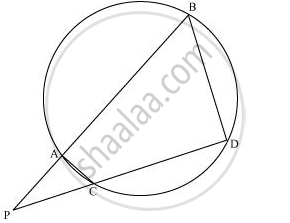SSC (Marathi Semi-English) 10thMaharashtra State Board
Share

# In the given figure, two chords AB and CD of a circle intersect each other at the point P (when produced) outside the circle. Prove that - SSC (Marathi Semi-English) 10th - Geometry

#### Question

In the given figure, two chords AB and CD of a circle intersect each other at the point P (when produced) outside the circle. Prove that

(i) ΔPAC ∼ ΔPDB

(ii) PA.PB = PC.PD#### Solution

(i) In ΔPAC and ΔPDB,

∠P = ∠P (Common)

∠PAC = ∠PDB (Exterior angle of a cyclic quadrilateral is ∠PCA = ∠PBD equal to the opposite interior angle)

∴ ΔPAC ∼ ΔPDB

(ii)We know that the corresponding sides of similar triangles are proportional.

∴ PA/PD = AC/DB = PC/PB

=>PA/PD = PC/PB

∴ PA.PB = PC.PD

Is there an error in this question or solution?

#### APPEARS IN

NCERT Solution for Mathematics Textbook for Class 10 (2019 to Current)
Chapter 6: Triangles
Ex. 6.60 | Q: 8 | Page no. 153
Solution In the given figure, two chords AB and CD of a circle intersect each other at the point P (when produced) outside the circle. Prove that Concept: Similarity of Triangles.
S